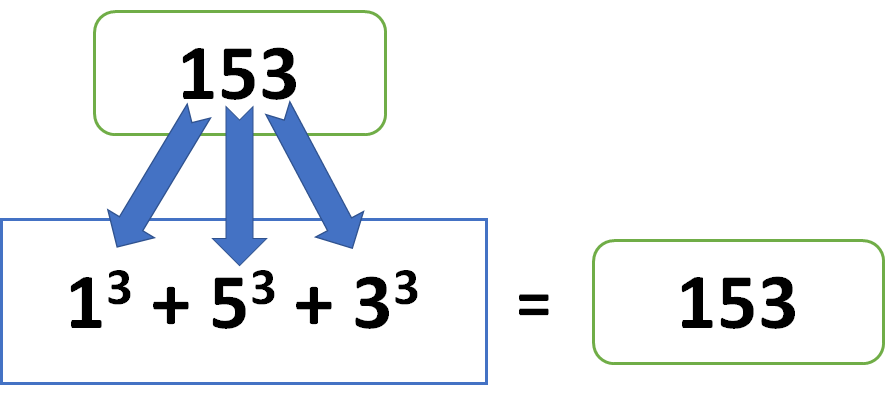Select Page

# Program to find Armstrong Number in ABAP

by | Jun 3, 2018 | ABAP Programs

Home » SAP » ABAP » ABAP Programs » Program to find Armstrong Number in ABAP

Preface – This post is part of the ABAP Programs series.

Sometimes, there is a need to know if the number provided by user is Armstrong number. In that case we program to find Armstrong Number in ABAP. It is not a question that is merely asked in an interview but an important keyword that is used in day to day programming.

## Introduction

Armstrong Number is a number whose sum of cube of individual number is equal to the entire number itself.

For example: 153 = 13 + 53 + 33

This can be achieved in ABAP with the help of loop in ABAP and truncate in ABAP. The given program implements the same:Armstrong Number – Image Illustration

## ABAP Program to find Armstrong Number

```PARAMETERS: lv_data1(10) type p.
Data: lv_digit(10) type i,
lv_final(1) type i,
lv_arm(10) type i,
lv_data2(10) type p.
lv_data2 = lv_data1.
while lv_data2 <> 0.
lv_digit = lv_data2 MOD 10.
lv_arm = lv_arm + ( lv_digit  * lv_digit  * lv_digit ).
lv_data2 = trunc( lv_data2 / 10 ).
ENDWHILE.
If lv_data1 EQ lv_arm.
Write: 'It is an Armstrong Number'.
Else.
Write: 'It is not an Armstrong Number'.
ENDIF.
```

## Explanation

In the program, mentioned above, we have written a mathematical algorithm. The above program is explained below, step by step:

1. Initially, we have defined a parameter lv_data1 of type i.e. packed (another form of float) and length 10. This parameter will be used to take the input.
2. Later, we have defined four variables: lv_digit, lv_final, lv_arm, lv_data2. These variables will be used in the algorithm discussed in further step.
3. To find if the number is Armstrong number or not, we will have to take out each individual number from the input. Then, get the summation of their cube.
4. If the summation achieved in above step is equal to the original number, then we print ‘It is an Armstrong Number’ else we print ‘It is not an Armstrong Number’.

## Author

•A Full Stack Developer with 10+ years of experience in technical content creation.

•